# RG Flows Entanglement Holography Strings 2013 Sogang Univesity

• Slides: 51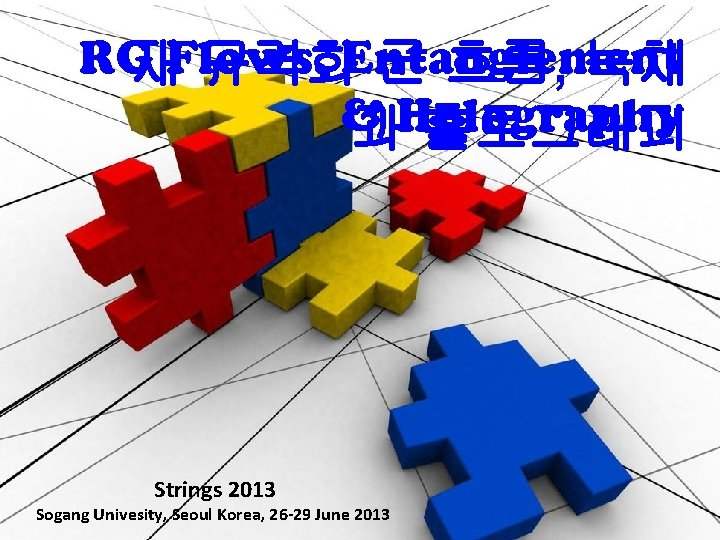RG 재Flows, 규격화Entanglement 군 흐름, 녹채 & Holography 과 홀로그래피 Strings 2013 Sogang Univesity, Seoul Korea, 26 -29 June 2013New Dialogues in Theoretical Physics: Particle Physics Statistical Mechanics RRenormalization Group Flows Quantum Field Theory Holographic RG Flows Many Body Theory Quantum Information Entanglement Condensed Matter Theory Holography String Theory Quantum Gravity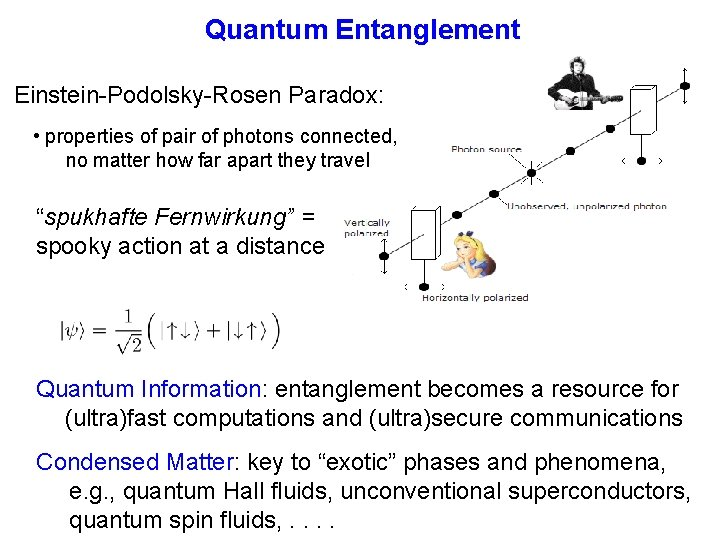Quantum Entanglement Einstein-Podolsky-Rosen Paradox: • properties of pair of photons connected, no matter how far apart they travel “spukhafte Fernwirkung” = spooky action at a distance Quantum Information: entanglement becomes a resource for (ultra)fast computations and (ultra)secure communications Condensed Matter: key to “exotic” phases and phenomena, e. g. , quantum Hall fluids, unconventional superconductors, quantum spin fluids, . .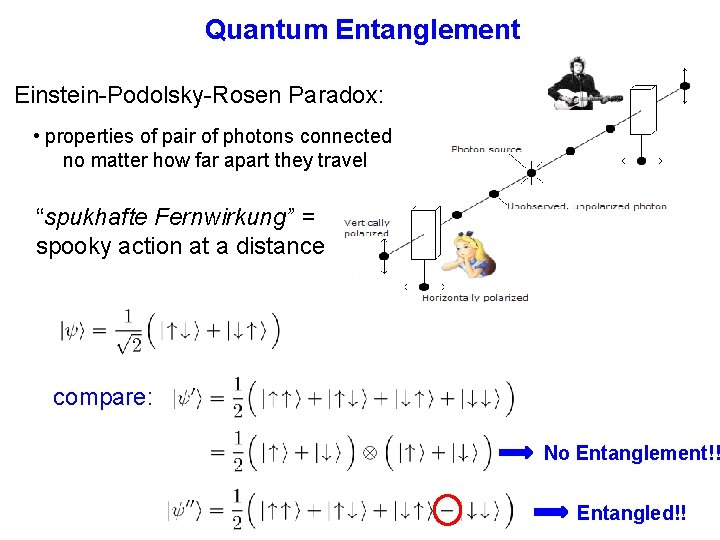Quantum Entanglement Einstein-Podolsky-Rosen Paradox: • properties of pair of photons connected no matter how far apart they travel “spukhafte Fernwirkung” = spooky action at a distance compare: No Entanglement!! Entangled!!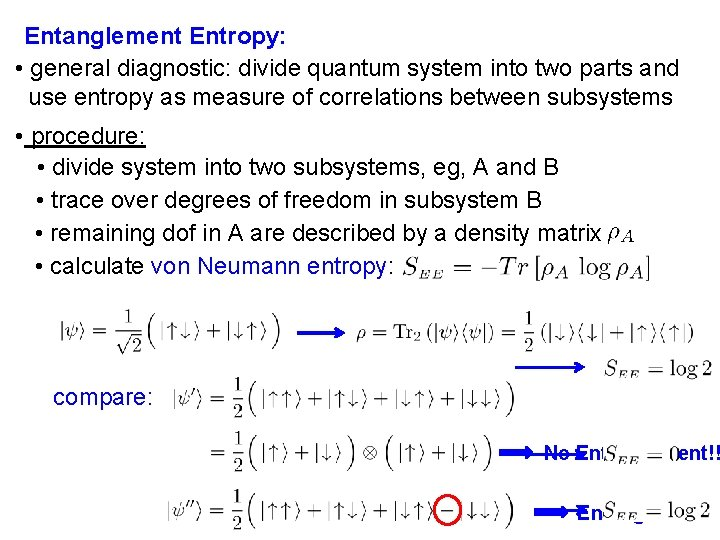Entanglement Entropy: • general diagnostic: divide quantum system into two parts and use entropy as measure of correlations between subsystems • procedure: • divide system into two subsystems, eg, A and B • trace over degrees of freedom in subsystem B • remaining dof in A are described by a density matrix • calculate von Neumann entropy: compare: No Entanglement!! Entangled!!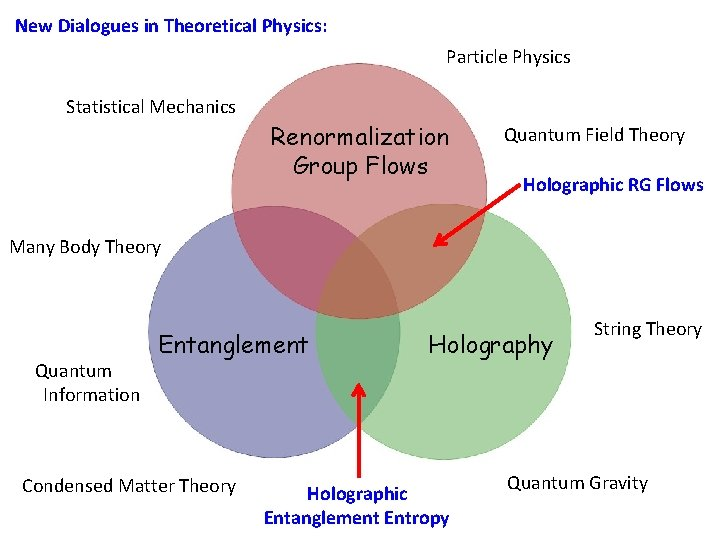New Dialogues in Theoretical Physics: Particle Physics Statistical Mechanics Renormalization Group Flows Quantum Field Theory Holographic RG Flows Many Body Theory Quantum Information Entanglement Condensed Matter Theory Holographic Entanglement Entropy String Theory Quantum GravityEntanglement Entropy 2: • in the context of holographic entanglement entropy, SEE is applied in the context of quantum field theory • in QFT, typically introduce a (smooth) boundary or entangling surface which divides the space into two separate regions • integrate out degrees of freedom in “outside” region • remaining dof are described by a density matrix calculate von Neumann entropy: B AEntanglement Entropy 2: • remaining dof are described by a density matrix calculate von Neumann entropy: B A R • result is UV divergent! dominated by short-distance correlations • must regulate calculation: = short-distance cut-off = spacetime dimension • careful analysis reveals geometric structure, eg,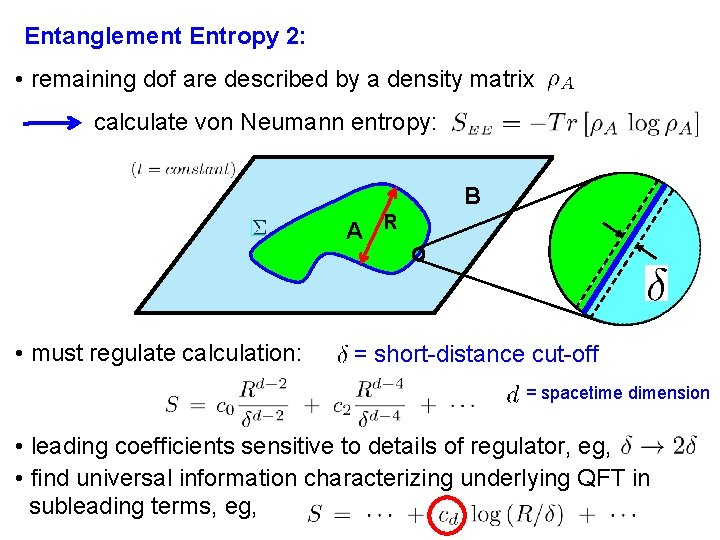Entanglement Entropy 2: • remaining dof are described by a density matrix calculate von Neumann entropy: B A R • must regulate calculation: = short-distance cut-off = spacetime dimension • leading coefficients sensitive to details of regulator, eg, • find universal information characterizing underlying QFT in subleading terms, eg,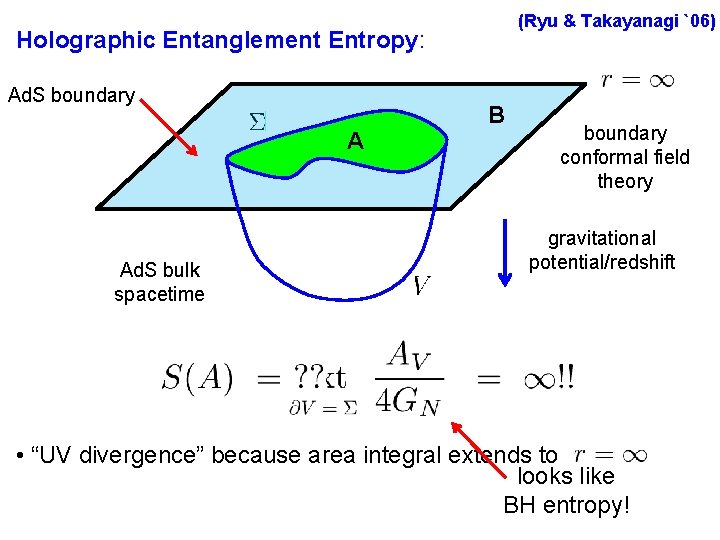(Ryu & Takayanagi `06) Holographic Entanglement Entropy: Ad. S boundary A Ad. S bulk spacetime B boundary conformal field theory gravitational potential/redshift • “UV divergence” because area integral extends to looks like BH entropy!(Ryu & Takayanagi `06) Holographic Entanglement Entropy: Ad. S boundary A B cut-off in boundary CFT: regulator surface • “UV divergence” because area integral extends to centralintroduce charge “regulator surface” at large radius: • as usual, (counts dof) short-distance cut-off in boundary theory: “Area Law”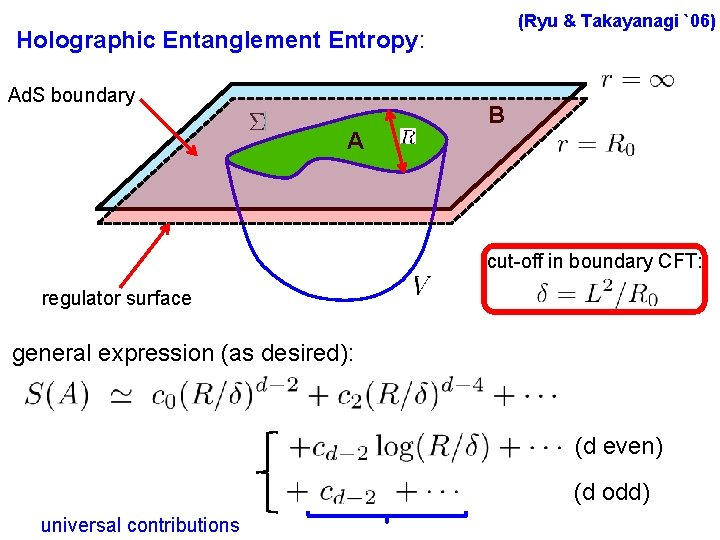(Ryu & Takayanagi `06) Holographic Entanglement Entropy: Ad. S boundary A B cut-off in boundary CFT: regulator surface general expression (as desired): (d even) (d odd) universal contributionsHolographic Entanglement Entropy: (Ryu & Takayanagi `06) conjecture Extensive consistency tests: 1) leading contribution yields “area law” 2) recover known results for d=2 CFT: 3) (Holzhey, Larsen & Wilczek) (Calabrese & Cardy) in a pure state and 4) for thermal bath: both yield same bulk surface V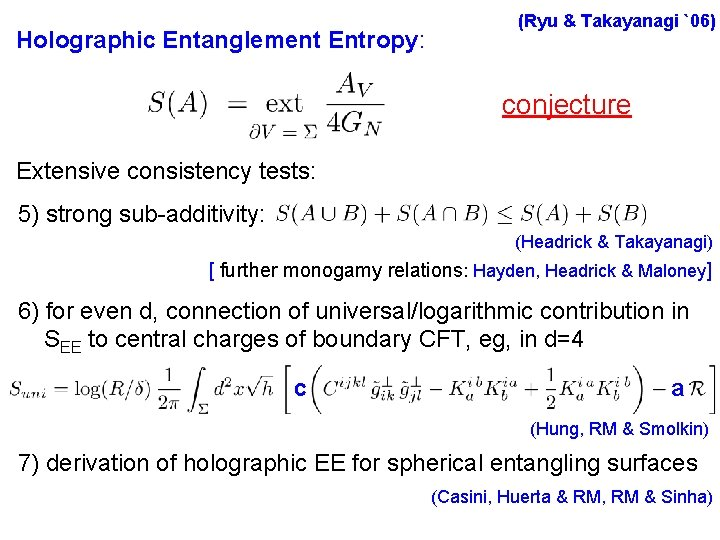Holographic Entanglement Entropy: (Ryu & Takayanagi `06) conjecture Extensive consistency tests: 5) strong sub-additivity: (Headrick & Takayanagi) [ further monogamy relations: Hayden, Headrick & Maloney] 6) for even d, connection of universal/logarithmic contribution in SEE to central charges of boundary CFT, eg, in d=4 c a (Hung, RM & Smolkin) 7) derivation of holographic EE for spherical entangling surfaces (Casini, Huerta & RM, RM & Sinha)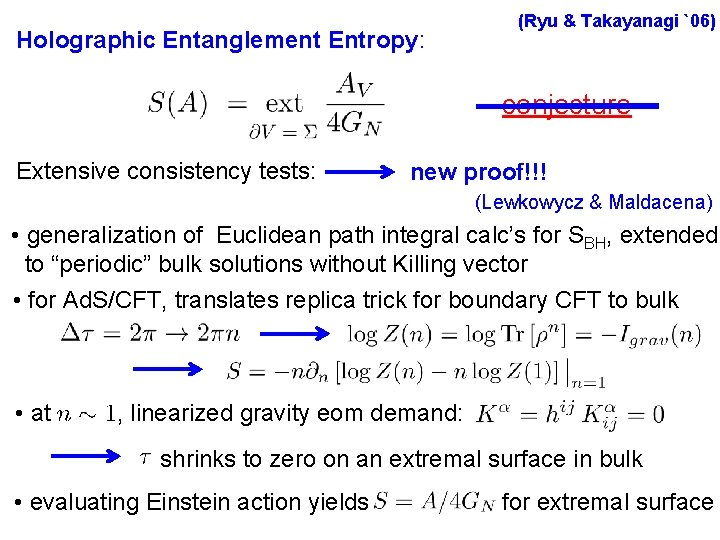Holographic Entanglement Entropy: (Ryu & Takayanagi `06) conjecture Extensive consistency tests: new proof!!! (Lewkowycz & Maldacena) • generalization of Euclidean path integral calc’s for SBH, extended to “periodic” bulk solutions without Killing vector • for Ad. S/CFT, translates replica trick for boundary CFT to bulk • at , linearized gravity eom demand: shrinks to zero on an extremal surface in bulk • evaluating Einstein action yields for extremal surface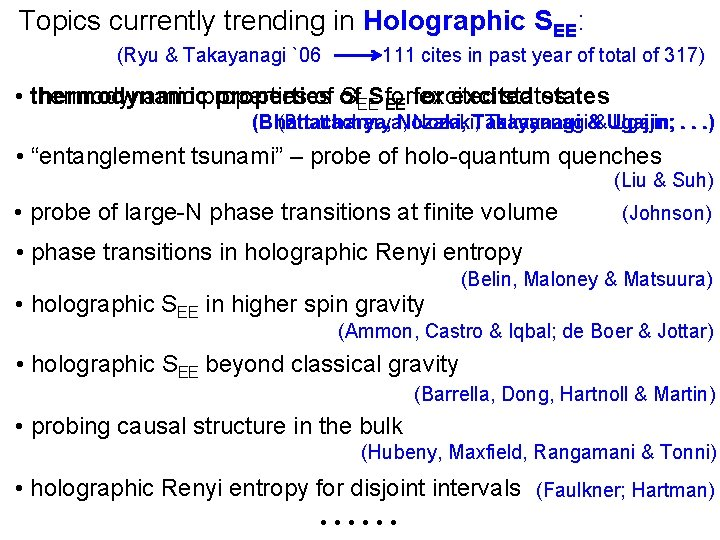Topics currently trending in Holographic SEE: (Ryu & Takayanagi `06 111 cites in past year of total of 317) • thermodynamic properties of of SEESfor excited states EE for (Bhattacharya, Nozaki, Takayanagi&&Ugajin; . . . ) (Bhattacharya, Nozaki, • “entanglement tsunami” – probe of holo-quantum quenches (Liu & Suh) • probe of large-N phase transitions at finite volume (Johnson) • phase transitions in holographic Renyi entropy • holographic SEE in higher spin gravity (Belin, Maloney & Matsuura) (Ammon, Castro & Iqbal; de Boer & Jottar) • holographic SEE beyond classical gravity (Barrella, Dong, Hartnoll & Martin) • probing causal structure in the bulk (Hubeny, Maxfield, Rangamani & Tonni) • holographic Renyi entropy for disjoint intervals (Faulkner; Hartman) ● ● ●New Dialogues in Theoretical Physics: Particle Physics Statistical Mechanics John Preskill (quant-ph/9904022) “Quantum information and physics: some future directions” ? ? Renormalization Group Flows Quantum Field Theory Holographic RG Flows Many Body Theory Quantum Information Entanglement Condensed Matter Theory Holographic Entanglement Entropy String Theory Quantum GravityZamolodchikov c-theorem (1986): • renormalization-group (RG) flows can seen as one-parameter motion in the space of (renormalized) coupling constants with beta-functions as “velocities” • for unitary, renormalizable QFT’s in two dimensions, there exists a positive-definite real function of the coupling constants : 1. monotonically decreasing along flows: 2. “stationary” at fixed points : : 3. at fixed points, it equals central charge of corresponding CFT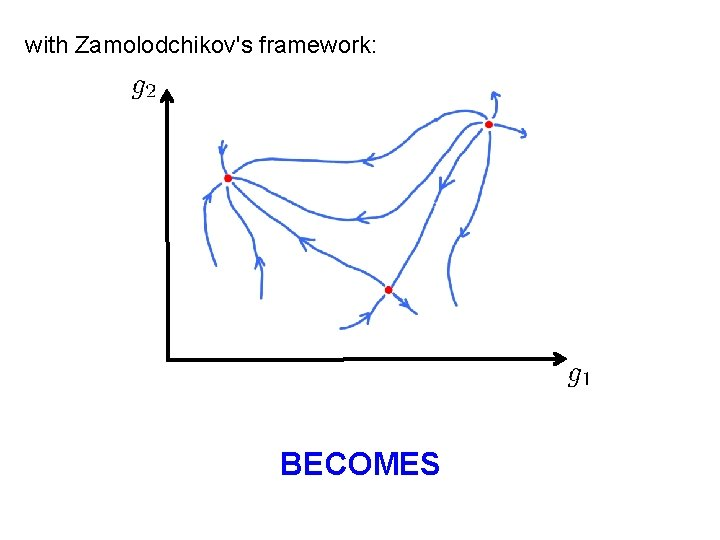with Zamolodchikov's framework: BECOMESwith Zamolodchikov's framework: ● ● Consequence for any RG flow in d=2: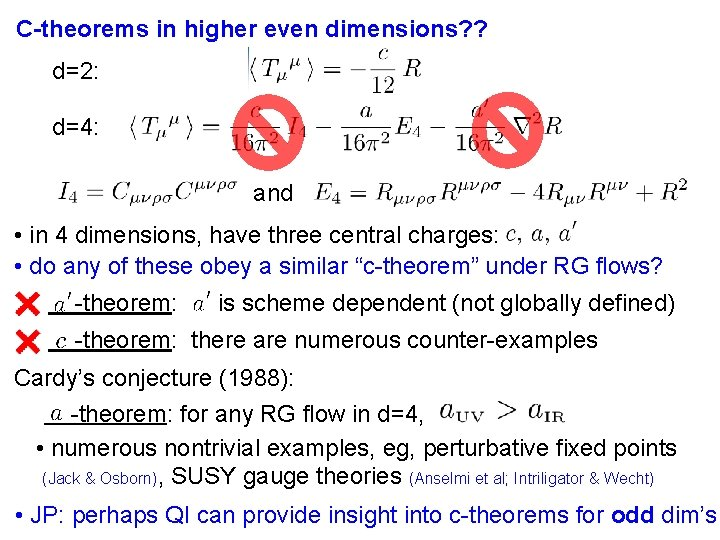C-theorems in higher even dimensions? ? d=2: d=4: and • in 4 dimensions, have three central charges: • do any of these obey a similar “c-theorem” under RG flows? -theorem: is scheme dependent (not globally defined) -theorem: there are numerous counter-examples Cardy’s conjecture (1988): -theorem: for any RG flow in d=4, • numerous nontrivial examples, eg, perturbative fixed points (Jack & Osborn), SUSY gauge theories (Anselmi et al; Intriligator & Wecht) • JP: perhaps QI can provide insight into c-theorems for odd dim’s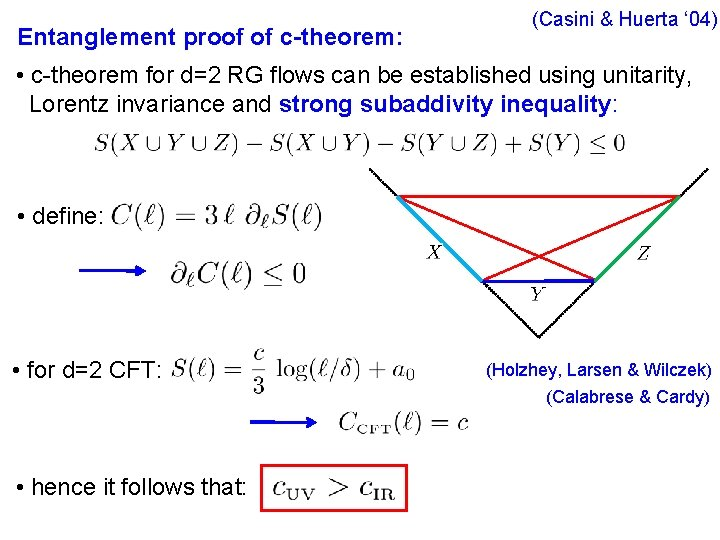Entanglement proof of c-theorem: (Casini & Huerta ‘ 04) • c-theorem for d=2 RG flows can be established using unitarity, Lorentz invariance and strong subaddivity inequality: • define: • for d=2 CFT: (Holzhey, Larsen & Wilczek) (Calabrese & Cardy) • hence it follows that:New Dialogues in Theoretical Physics: Particle Physics Statistical Mechanics c theorems? ? Renormalization Group Flows Quantum Field Theory Holographic RG Flows Many Body Theory Quantum Information Entanglement Condensed Matter Theory Holographic Entanglement Entropy String Theory Quantum Gravity(Girardello, Petrini, Porrati and Zaffaroni, hep-th/9810126) (Freedman, Gubser, Pilch & Warner, hep-th/9904017) Holographic RG flows: • imagine potential has stationary points giving negative Λ • consider metric: • at stationary points, Ad. S 5 vacuum: with • HRG flow: solution starts at one stationary point at large radius and ends at another at small radius – connects CFTUV to CFTIR ● ● UV IR ●(Girardello, Petrini, Porrati and Zaffaroni, hep-th/9810126) (Freedman, Gubser, Pilch & Warner, hep-th/9904017) Holographic RG flows: • for general flow solutions, define: Einstein equations null energy condition • at stationary points, and hence • using holographic trace anomaly: (e. g. , Henningson & Skenderis) supports Cardy’s conjecture • for Einstein gravity, central charges equal :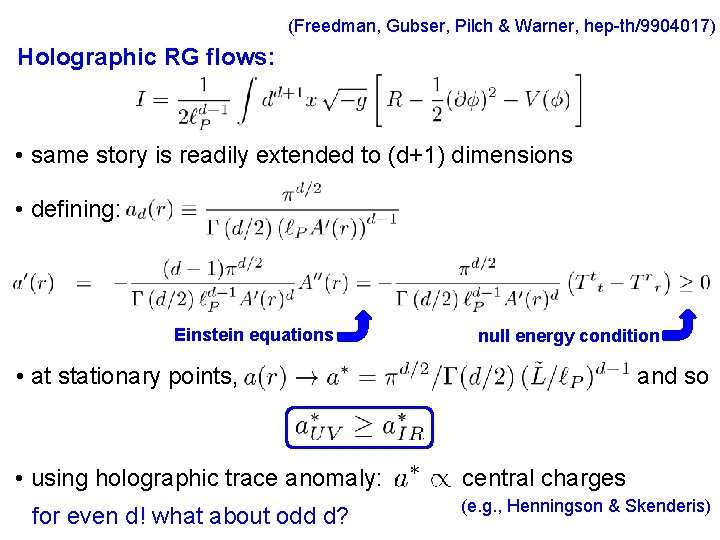(Freedman, Gubser, Pilch & Warner, hep-th/9904017) Holographic RG flows: • same story is readily extended to (d+1) dimensions • defining: Einstein equations null energy condition • at stationary points, • using holographic trace anomaly: for even d! what about odd d? and so central charges (e. g. , Henningson & Skenderis)Improved Holographic RG Flows: • add higher curvature interactions to bulk gravity action provides holographic field theories with, eg, so that we can clearly distinguish evidence of a-theorem (Nojiri & Odintsov; Blau, Narain & Gava) • construct “toy models” with fixed set of higher curvature terms (where we can maintain control of calculations) What about the swampland? • constrain gravitational couplings with consistency tests (positive fluxes; causality; unitarity) and use best judgement • ultimately one needs to fully develop string theory for interesting holographic backgrounds! • “if certain general characteristics are true for all CFT’s, then holographic CFT’s will exhibit the same features”Toy model: (RM & Robinsion; RM, Paulos & Sinha) with • three dimensionless couplings: • again, gravitational eom and null energy conditon yield: (RM & Sinha) where with central charge of boundary CFT • toy model supports for Cardy’s conjecture in four dimensions(RM & Sinha) • for holographic RG flows with general d, find: where with • trace anomaly for CFT’s with even d: (Deser & Schwimmer) • verify that we have precisely reproduced central charge (Henningson & Skenderis; Nojiri & Odintsov; Blau, Narain & Gava; Imbimbo, Schwimmer, Theisen & Yankielowicz) agrees with Cardy’s conjecture What about odd d? ?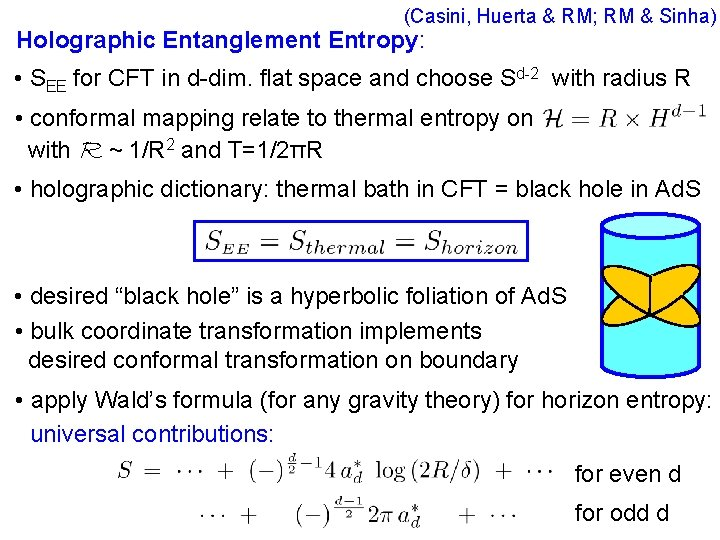(Casini, Huerta & RM; RM & Sinha) Holographic Entanglement Entropy: • SEE for CFT in d-dim. flat space and choose Sd-2 with radius R • conformal mapping relate to thermal entropy on with R ~ 1/R 2 and T=1/2πR • holographic dictionary: thermal bath in CFT = black hole in Ad. S • desired “black hole” is a hyperbolic foliation of Ad. S • bulk coordinate transformation implements desired conformal transformation on boundary • apply Wald’s formula (for any gravity theory) for horizon entropy: universal contributions: for even d for odd dC-theorem conjecture: (RM & Sinha) • identify central charge with universal contribution in entanglement entropy of ground state of CFT across sphere Sd-2 of radius R: for even d for odd d (any gravitational action) • for RG flows connecting two fixed points (“unitary” models) unified framework to consider c-theorem for odd or even d connect to Cardy’s conjecture: for any CFT in even d(Jafferis, Klebanov, Pufu & Safdi) F-theorem: • examine partition function for broad classes of 3 -dimensional quantum field theories on three-sphere (SUSY gauge theories, perturbed CFT’s & O(N) models) • in all examples, F= – log Z(S 3)>0 and decreases along RG flows conjecture: • also naturally generalizes to higher odd d • coincides with entropic c-theorem (Casini, Huerta & RM) • focusing on renormalized or universal contributions, eg, • generalizes to general odd d: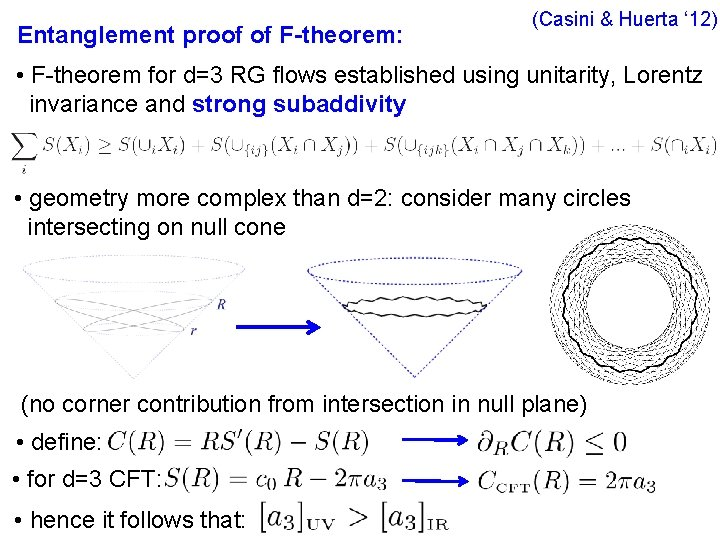Entanglement proof of F-theorem: (Casini & Huerta ‘ 12) • F-theorem for d=3 RG flows established using unitarity, Lorentz invariance and strong subaddivity • geometry more complex than d=2: consider many circles intersecting on null cone (no corner contribution from intersection in null plane) • define: • for d=3 CFT: • hence it follows that:(Liu & Mezei) “Renormalized” Entanglement Entropy: • SEE is UV divergent, so must take care in defining universal term • divergences determined by local geometry of entangling surface with covariant regulator, eg, • can isolate finite term with appropriate manipulations, eg, d=3: c-function of d=4: • unfortunately, holographic experiments indicate good c-functions for d>3 Casini & Huerta are not(Casini, Huerta, RM & Yale) “Renormalized” Entanglement Entropy 2: • SEE is UV divergent, so must take care in defining universal term • mutual information is intrinsically finite and so offers alternative approach to regulate SEE • with and • choice ensures that a 3 is not polluted by UV fixed point • naturally extends to defining ad in higher odd dimensions • for d=3, entropic proof of F-theorem can be written in terms of mutual information(Komargodski & Schwimmer; see also: Luty, Polchinski & Rattazzi) a-theorem and Dilaton Effective Action (Schwimmer & Theisen) • analyze RG flow as “broken conformal symmetry” • couple theory to “dilaton” (conformal compensator) and organize effective action in terms of diffeo X Weyl invariant: • follow effective dilaton action to IR fixed point, eg, : ensures UV & IR anomalies match • with , only contribution to 4 pt amplitude with null dilatons: • dispersion relation plus optical theorem demand: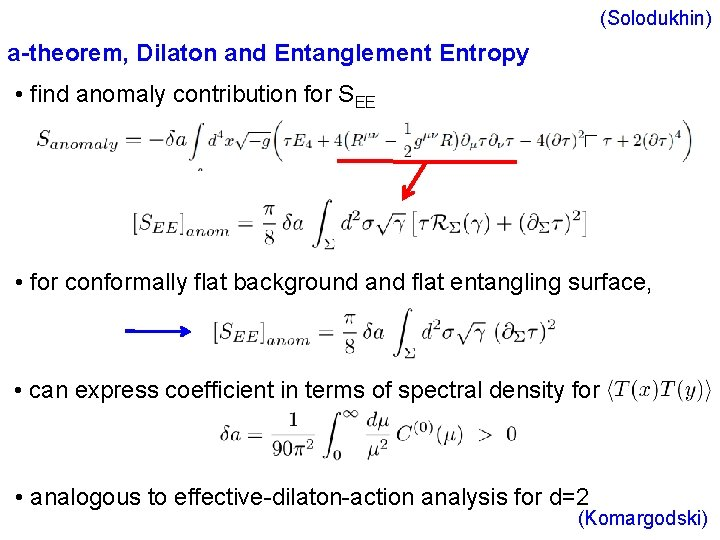(Solodukhin) a-theorem, Dilaton and Entanglement Entropy • find anomaly contribution for SEE • for conformally flat background and flat entangling surface, • can express coefficient in terms of spectral density for • analogous to effective-dilaton-action analysis for d=2 (Komargodski)Questions: • how much of Zamalodchikov’s structure for d=2 RG flows extends higher dimensions? d=3 entropic c-function not always stationary at fixed points (Klebanov, Nishioka, Pufu & Safdi) • can c-theorems be proved for higher dimensions? eg, d=5 or 6 dilaton-effective-action would require subtle refinement for d=6 (Elvang, Freedman, Hung, Kiermaier, RM & Theisen; Elvang & Olson) • does scale invariance imply conformal invariance beyond d=2? at least, perturbatively in d=4 (Nakayama) (Luty, Polchinski & Rattazzi) • further lessons for RG flows and entanglement from holography? translation of “null energy condition” to boundary theory? • what can entanglement entropy/quantum information really say about renormalization group and holography?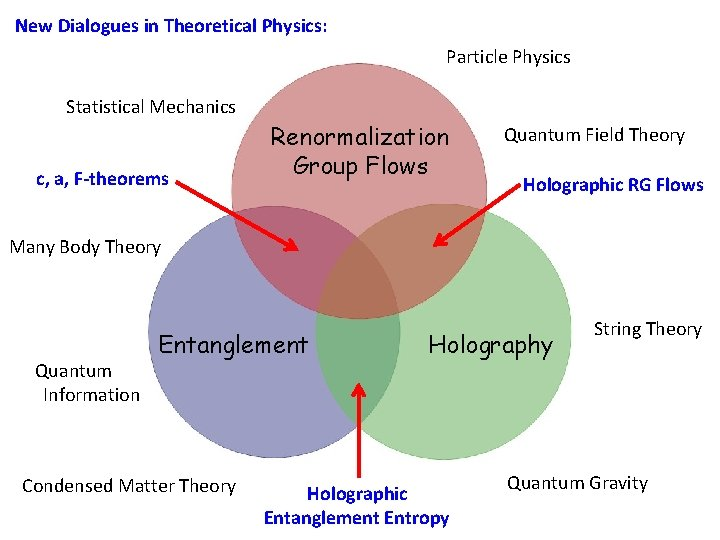New Dialogues in Theoretical Physics: Particle Physics Statistical Mechanics c, a, F-theorems Renormalization Group Flows Quantum Field Theory Holographic RG Flows Many Body Theory Quantum Information Entanglement Condensed Matter Theory Holographic Entanglement Entropy String Theory Quantum Gravity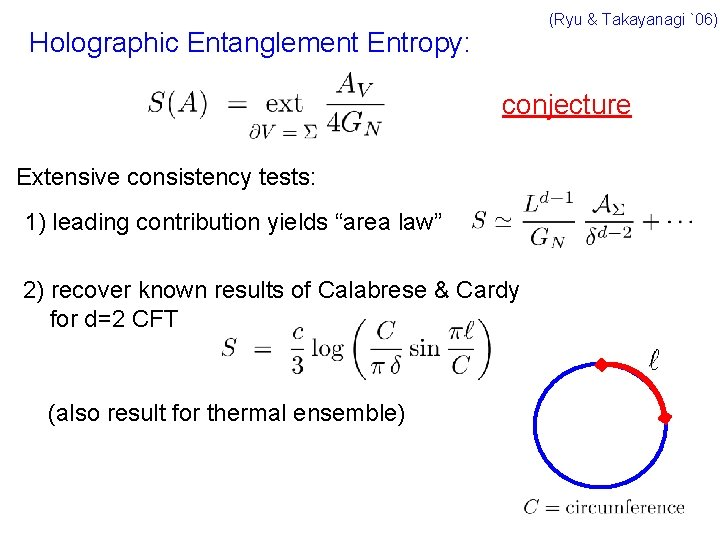(Ryu & Takayanagi `06) Holographic Entanglement Entropy: conjecture Extensive consistency tests: 1) leading contribution yields “area law” 2) recover known results of Calabrese & Cardy for d=2 CFT (also result for thermal ensemble)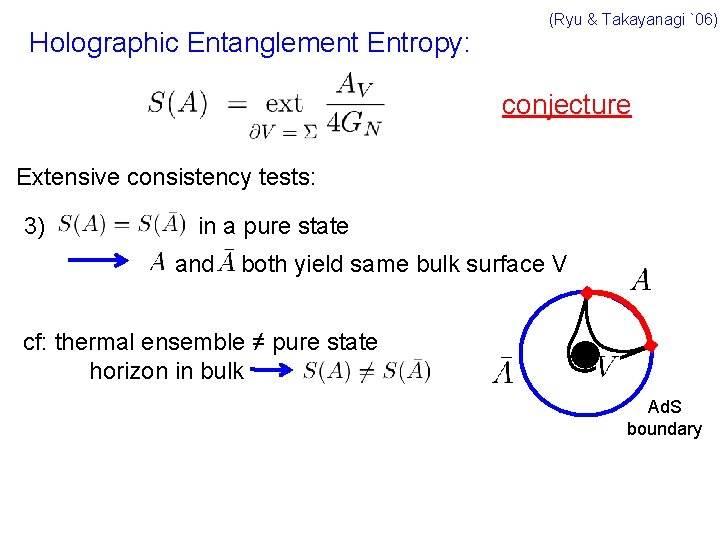Holographic Entanglement Entropy: (Ryu & Takayanagi `06) conjecture Extensive consistency tests: 3) in a pure state and both yield same bulk surface V cf: thermal ensemble ≠ pure state horizon in bulk Ad. S boundary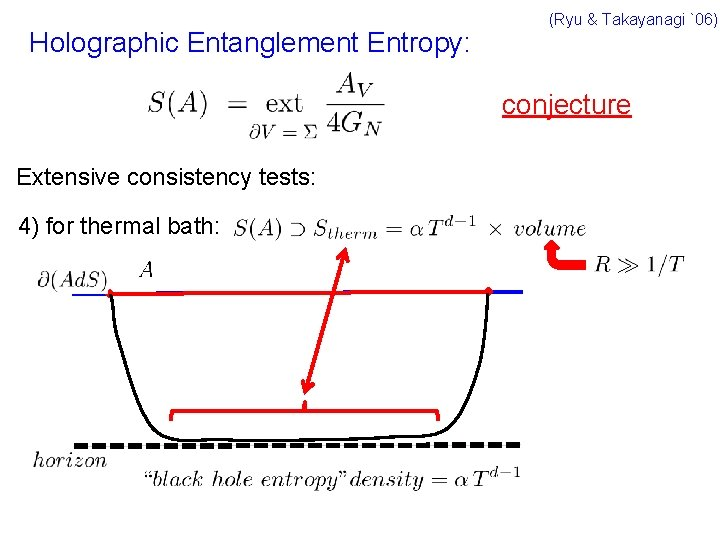Holographic Entanglement Entropy: (Ryu & Takayanagi `06) conjecture Extensive consistency tests: 4) for thermal bath:(Ryu & Takayanagi `06) Holographic Entanglement Entropy: conjecture Extensive consistency tests: 4) Entropy of eternal black hole = entanglement entropy of boundary CFT & thermofield double (Maldacena; Headrick) thermofield double boundary CFT extremal surface = bifurcation surface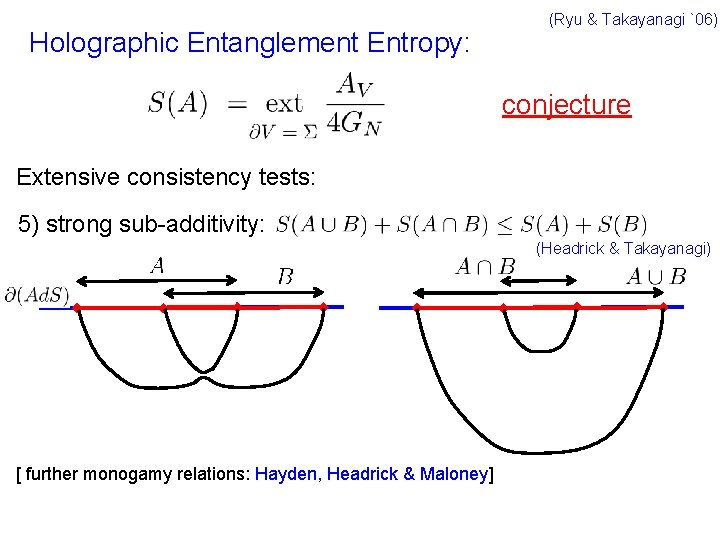Holographic Entanglement Entropy: (Ryu & Takayanagi `06) conjecture Extensive consistency tests: 5) strong sub-additivity: (Headrick & Takayanagi) [ further monogamy relations: Hayden, Headrick & Maloney]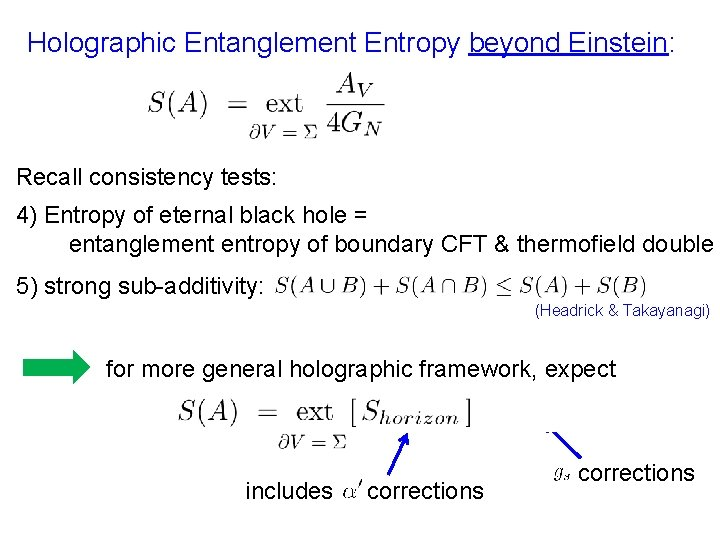Holographic Entanglement Entropy beyond Einstein: Recall consistency tests: 4) Entropy of eternal black hole = entanglement entropy of boundary CFT & thermofield double 5) strong sub-additivity: (Headrick & Takayanagi) for more general holographic framework, expect includes corrections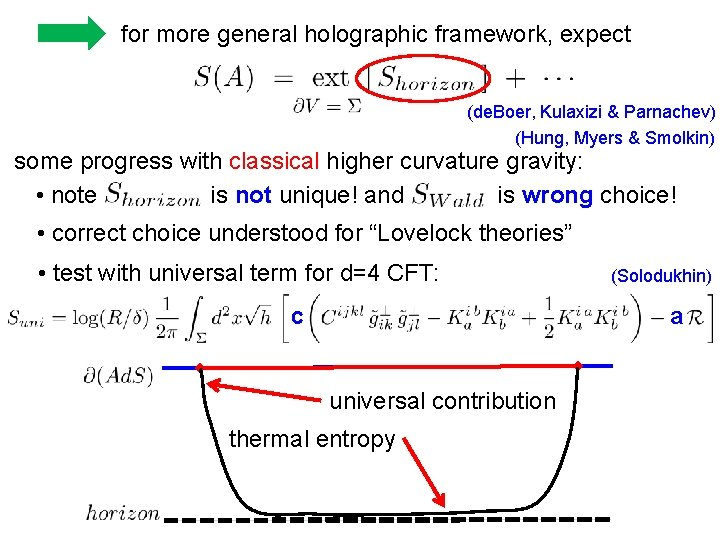for more general holographic framework, expect (de. Boer, Kulaxizi & Parnachev) (Hung, Myers & Smolkin) some progress with classical higher curvature gravity: • note is not unique! and is wrong choice! • correct choice understood for “Lovelock theories” • test with universal term for d=4 CFT: (Solodukhin) a c universal contribution thermal entropy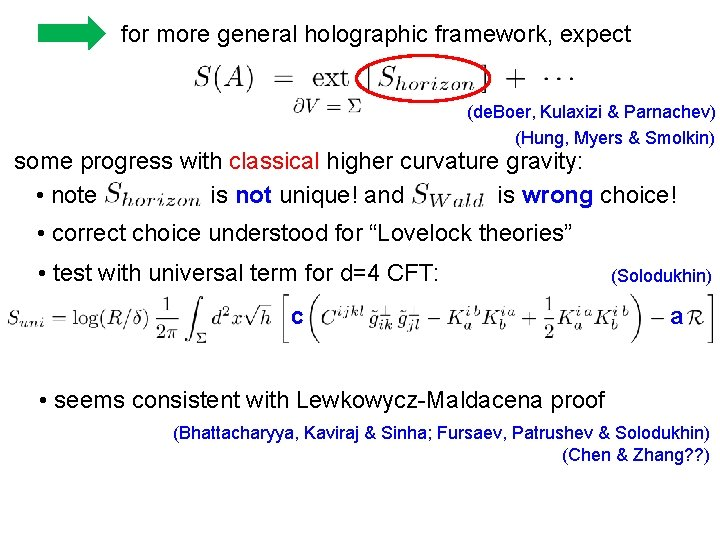for more general holographic framework, expect (de. Boer, Kulaxizi & Parnachev) (Hung, Myers & Smolkin) some progress with classical higher curvature gravity: • note is not unique! and is wrong choice! • correct choice understood for “Lovelock theories” • test with universal term for d=4 CFT: c (Solodukhin) a • seems consistent with Lewkowycz-Maldacena proof (Bhattacharyya, Kaviraj & Sinha; Fursaev, Patrushev & Solodukhin) (Chen & Zhang? ? )Lessons from Holographic EE: • compare two theories: • tune: both theories have same Ad. S vacuum • clearly SEE is not tied to causal structure or even geometry alone A boundary of causal domain extremal surface for GB gravity extremal surface for Einstein gravity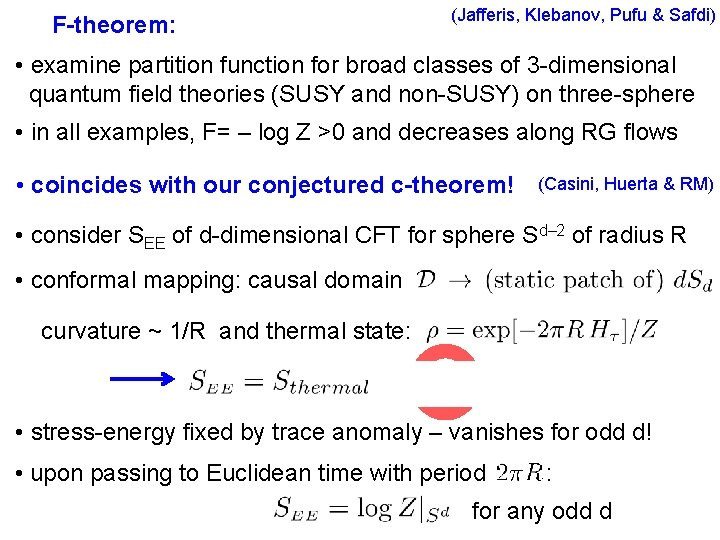F-theorem: (Jafferis, Klebanov, Pufu & Safdi) • examine partition function for broad classes of 3 -dimensional quantum field theories (SUSY and non-SUSY) on three-sphere • in all examples, F= – log Z >0 and decreases along RG flows • coincides with our conjectured c-theorem! (Casini, Huerta & RM) • consider SEE of d-dimensional CFT for sphere Sd– 2 of radius R • conformal mapping: causal domain curvature ~ 1/R and thermal state: • stress-energy fixed by trace anomaly – vanishes for odd d! • upon passing to Euclidean time with period : for any odd dF-theorem: • must focus on renormalized or universal contributions, eg, • generalizes to general odd d: • equivalence shown only for fixed points but good enough: UV IR • evidence for F-theorem (SUSY, perturbed CFT’s & O(N) models) supports present conjecture and our holographic analysis provides additional support for F-theorem